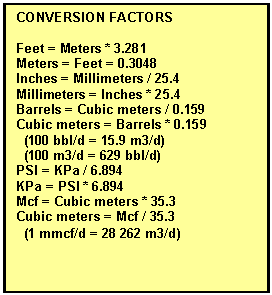CALCULATING fLuid Properties
The log analysis results can be transformed into reserves if the fluid properties are known or can be estimated. Use measured values if available, otherwise try these US DOE correlations.

STEP 1: Calculate fluid properties

1: PF = KP1 * DEPTH

2: PS = KP2

Where:  KP1 = 0.46 psi/foot for English units (use measured pressures where available)

KP1 = 10.4 KPa/meter for Metric units

KP2 = 14.7 to 600 psi for English units

KP2 = 100 to 4000 KPa for Metric units

3: DENShy = 8829.6 / (131.5 + API) / 62.4 * KD2

Where:  KD2 = 1.00 for English units

KD2 = 1000 for Metric units

.
4A: SGx = SGd * (1.0 + 0.00005912 * API * FT * log(PF / 114.7))
4B: GOR = (KG1 * SGx * PF ^ KG2) * EXP ((KG3 * API) / (FT + KT2))

Where:  KG1 = 0.0362 for API < 30

KG1 = 0.0178 for API > 30

KG2 = 1.0937 for API < 30

KG2 = 1.1870 for API > 30

KG3 = 25.7242 for API < 30

KG3 = 23.9318 for API > 30

KT2 = 460'F
SGd = gas specific gravity downhole (unitless)
SGx = gas specific gravity at 100psi (unitless)

NOTE: PF is in psi and FT in Fahrenheit for Equation 4.

5: Bo = 1 + KC1 * GOR * (KC2 + KC3 * GOR) * (FT – 60) * (API / DENShy)Where:  KC1 = 0.000 467 700 for API < 30

KC1 = 0.000 467 000 for API > 30

KC2 = 0.000 017 510 for API < 30

KC2 = 0.000 011 000 for API > 30

KC3 = 0.000 000 018 for API < 30

KC3 = 0.000 000 0013 for API > 30

NOTE: DENShy is in gm/cc and FT in Fahrenheit for Equation 5.  GOR is in scf/bbl.

6: Bg =  (PS * (TF + KT2)) / (PF * (TS + KT2)) * ZF

Where:  KT2 = 460 for English units

KT2 = 273 for Metric units

7: VISd = 10^(10^(3.0324 – 0.02023 * API) / (FT^1.163)) – 1

8: VISo = (10.715 / (GOR +100) ^ 0.515) *  VISd
^ (5.44 / (GOR + 150) ^ 0.338)

Where:
Bg = gas formation volume factor (fractional)
Bo = oil formation volume factor (fractional(
DEPTH = formation depth (ft or m)
GOR = gas oil ratio (ocf/bbl or m3/m3)
PF = formation pressure (psi or KPa)
PS = standard surface pressure (psi or KPa)
TF = formation temperature (degrees C or degrees F)
TS = standard surface temperature (degrees C or degrees F)COMMENTS:
These fluid properties equations are derived from a US Department of Energy publication. Measured values should be used if available.

Page Views ---- Since 01 Jan 2015
Copyright 2023 by Accessible Petrophysics Ltd.
CPH Logo, "CPH", "CPH Gold Member", "CPH Platinum Member", "Crain's Rules", "Meta/Log", "Computer-Ready-Math", "Petro/Fusion Scripts" are Trademarks of the Author